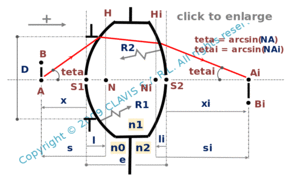and orgeometrical optics
paraxial conjugation

# paraxial conjugation by a thick lens interfacing different media

Caution
Fill in all input cells.
Choose all parameters unit.
n0, n1 and n2 can be either values or references of glasses from the catalog. If values only are entered, the wavelength will not be considered.
e is positive.
Raddii and x are algebric or infinite. Enter "infinite" for a plane surface or an object at infinity.
Positive direction is according the horizontal arrow in the bottom right of the scheme.

InputValueunit
n0
n2
n1
R1
R2
e
x
OutputValueUnit
lto be calculated
lito be calculated
sto be calculated
sito be calculated
xito be calculated
mto be calculatedn0 : refraction index of the first medium n2 : refraction index of the second medium n1 : refraction index of the lens R1 : radius of curvature of the first surface R2 : radius of curvature of the second surface x : distance from the vertex of the first surface to the object l : distance from the vertex of the first surface to the primary principal plane li : distance from the vertex of the second surface to the secondary principal plane s : distance from the primary principal plane to the object si : distance from the secondary principal plane to the image xi : distance from the vertex of the second surface to the image

Note
This calculation page can not detect all unrealistic inputs.